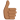# Find acceleration and speed of a box

## Homework Statement

A large cardboard box of mass 0.75 kg is pushed across a horizontal floor by a force of 4.5 N. The motion of the box is opposed by (i) a frictional force of 1.5 N between the box and the floor, and (ii) an air resistance force kv2, where k = 6 * 10-2 kg m-1 and v is the speed of the box in m s-1.

Sketch a diagram showing the directions of the forces which act on the moving box. Calculate maximum values for (a) the acceleration of the box, (b) its speed.

Answers: (a) 4.0 m s-2, (b) 7.1 m s-1

2. The attempt at a solution
While I do get the correct answers, I am a bit confused.

(a) F = ma
4.5 - 1.5 = 0.75 * a
a = 4 m s-2

(b) 4.5 = 1.5 + kv2
3 = 6 * 10-2 * v2
v = 7.1 m s-1

My confusion is the following: if 4.5 N which is moving "forward" equals to 1.5 N and the air resistance force kv2 how does the box actually move? Shouldn't it stand still? I known that a body might be in equilibrium and can move (e. g. a brick on a rope spun in a circle) but not sure how to apply it to this situation (whether this applies at all). And if it is equal, then why not 4.5 - 1.5 - kv2 = 0.75 * a?

Maybe my graph is wrong and that is why I don't see the situation in the right way. Or maybe my calculations are wrong and I luckily got a similar answer.Thanks in advance for any help!

Related Introductory Physics Homework Help News on Phys.org
Doc Al
Mentor
My confusion is the following: if 4.5 N which is moving "forward" equals to 1.5 N and the air resistance force kv2 how does the box actually move? Shouldn't it stand still?
Once the net force equals zero, the box will no longer accelerate. But it will keep moving at whatever speed it has attained.

•moenste
Once the net force equals zero, the box will no longer accelerate. But it will keep moving at whatever speed it has attained.
Thank you Doc Al. That explains (b) and in that case I think that my graph is correct (right?).

But why don't we use the air resistance force in (a)?

Doc Al
Mentor
That explains (b) and in that case I think that my graph is correct (right?).
Your diagram is just missing the normal force from the floor, but otherwise it is fine.

But why don't we use the air resistance force in (a)?
Because you want the maximum acceleration, which is when the net force is maximum. As the box gains speed, the air resistance increases and the net force (and acceleration) decreases. The air resistance is least when the box first starts out and the speed is zero.

•moenste
Your diagram is just missing the normal force from the floor, but otherwise it is fine.
Ah, yes. This one: normal reaction which is directed north (just like http://img1.mnimgs.com/img/shared/discuss_editlive/2191102/2012_10_31_20_34_28/image3293772999898041151.jpg [Broken]).

Because you want the maximum acceleration, which is when the net force is maximum. As the box gains speed, the air resistance increases and the net force (and acceleration) decreases. The air resistance is least when the box first starts out and the speed is zero.
Now I get it. The question is "maximum value of the acceleration", therefore we have two constant forces (4.5 and 1.5) and only one which is dependent on speed (air res.). Therefore, if we need "maximum" we calculate using 4.5 N and 1.5 N when the speed is zero and air res. is zero aswell.

I think that's it. Thank you :).

Last edited by a moderator:
Doc Al
Mentor
Ah, yes. This one: normal reaction which is directed north (just like http://img1.mnimgs.com/img/shared/discuss_editlive/2191102/2012_10_31_20_34_28/image3293772999898041151.jpg [Broken]).
Right. (Though I would call it "up", not "north".)

Now I get it. The question is "maximum value of the acceleration", therefore we have two constant forces (4.5 and 1.5) and only one which is dependent on speed (air res.). Therefore, if we need "maximum" we calculate using 4.5 N and 1.5 N when the speed is zero and air res. is zero aswell.
Exactly. You've got it.Last edited by a moderator:
•moenste